# 8 and 9-Digit Subtraction

## NUMBERS AND OPERATIONSSubtraction is an arithmetic operation that is represented by a "minus" sign (-) and symbolizes the operation of removing objects from a collection.

It fulfills the property of the neutral element:

Any natural number subtracted by the number zero (0) results in the same number; for example: 8 - 0 = 8

It fulfills the distributive property:

The result of the subtraction of two numbers (A and B) multiplied by a third number (C) will be equivalent to the subtraction of their products (A x C - B x C); for example: 2 x (6 - 3) = 2 x 6 - 2 x 3

Subtraction is anti-commutative

A modification in the order of its elements (minuend and subtrahend) will modify the sign of the answer; for example: 6 - 4 ≠ 4 - 6.

Subtraction is non-associative

When three or more numbers are subtracted, the order in which the subtraction is performed always influences; for example: 5 - (3 - 2) ≠ (5 - 3) - 2.

#### Properly place the terms of the subtraction in natural numbers up to 999,999,999

What is the "minuend", the "subtrahend" and the "difference" in a subtraction?

- Minuend: Represents the total collection or initial set of elements.

- Subtrahend: Symbolizes the number or quantity of elements in which the minuend will be reduced.

- Difference or Rest: It corresponds to the unique set formed by separating or reducing the amount of the subtrahend on the minuend.

SPANISHENGLISH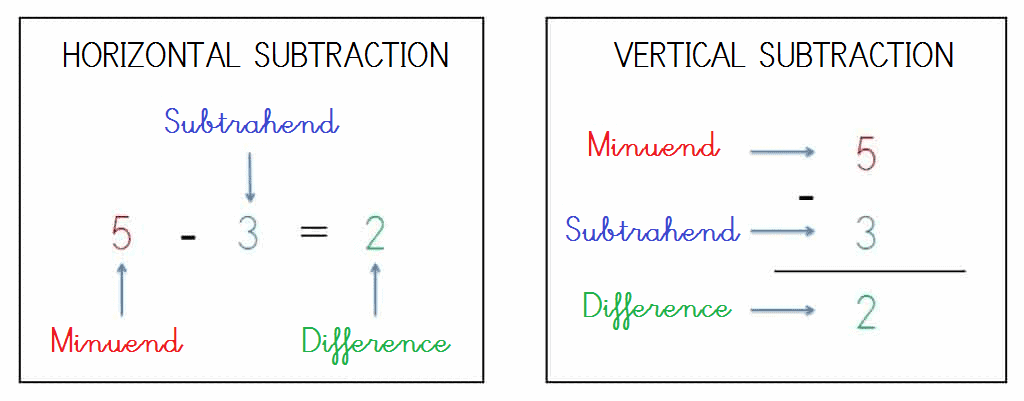How to build the algorithm of a vertical subtraction?

Next you will learn to construct vertical subtractions placing the symbols "-" e "=" and each of its terms (minuend, subtrahend and difference) in the corresponding place practicing with the different printable educational worksheets that we have elaborated for the natural numbers from 0 to 1,000,000,000 following the method based on vertical columns.

Remember, with the 8-digit and 9-digit subtractions you must always align, according to their place value, the ones (1s), the tens (10s), the hundreds (100s), the thousands (1,000s), the ten thousands (10,000s), the hundred thousands (100,000s) the millions (1,000,000s), the ten millions (10,000,000s) and the hundred millions (100,000,000s) before solving any subtraction operations.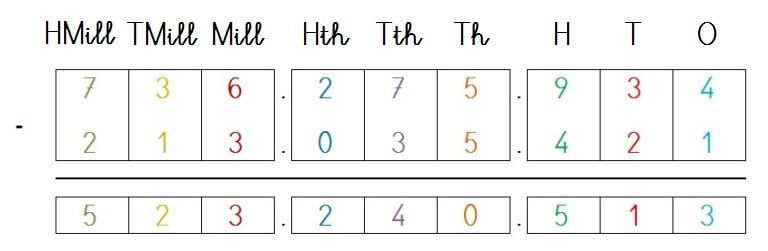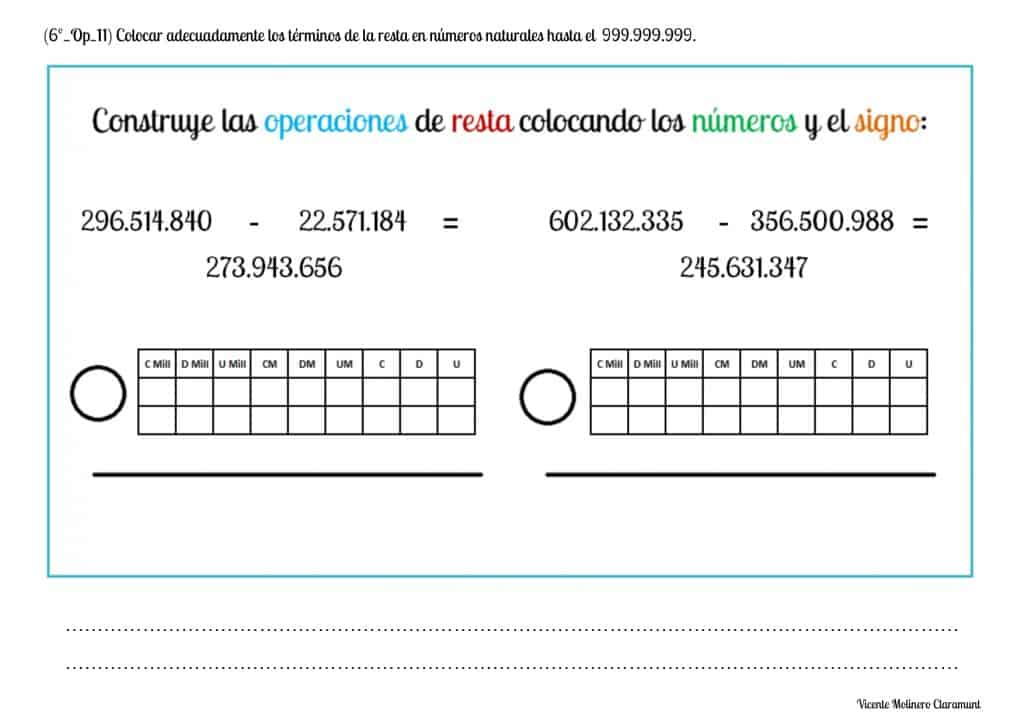#### Solve subtractions of two elements vertically without regrouping with whole numbers up to 999,999,999

How to subtract numbers up to nine digits without borrowing?

We present a solved example as well as some printable PDF activities accompanied by their explanation so that you can easily learn how to subtract in vertical and without regrouping eight and nine-digit numbers between 0 and the 1,000,000,000.

SPANISHENGLISH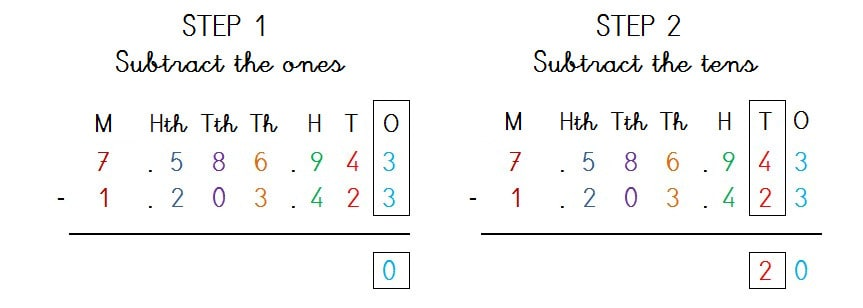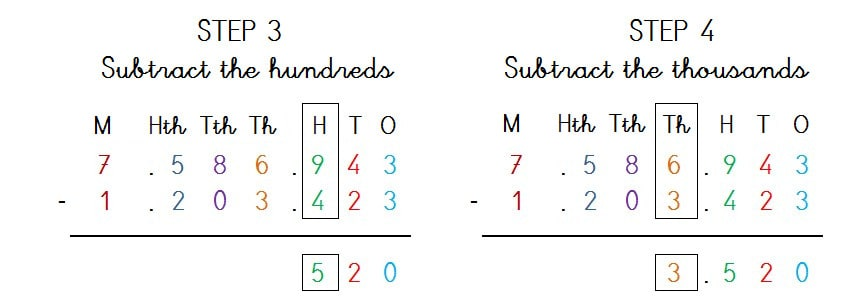#### Solve subtractions of two elements vertically with regrouping with whole numbers up to 999,999,999

Practice the 8 and 9-digits subtraction by borrowing with the whole numbers from 0 to 1,000,000,000 from our printable worksheets and become a mathematical expert.

We are going to explain how to solve any two-digit subtraction by regrouping using the the most taught methods in school: Austrian and American Method.

SPANISHENGLISHSUBTRACT BY COMPENSATION

The Austrian Method (also called "by compensation") proposes the resolution of subtractions with borrowing "adding units to both the minuend and the subtrahend" when the minuend figure is less than the digit of the subtrahend:1st) Can we subtract 3 - 7 in the ones column?

No, because 3 is less than 7 ( 3 < 7 ), therefore, we must ask for help in the tens column.

2nd) We add 1 ten to the subtrahend figure and 10 units to the minuend figure to compensate by equivalence the place value of its figures:

Subtrahend:

1 ten + 1 ten = 2 tens

Minuend:

3 ones + 10 ones (1 ten) = 13 ones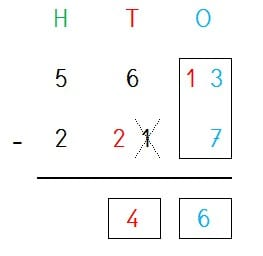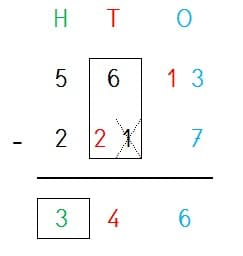3rd) We subtract the ones column because 13 is greater than 7:

13 - 7 = 6

4th) Next, we will subtract the tens column without having to borrow from the hundreds because 6 is greater than 2:

6 - 2 = 4

5th) And finally, we will subtract the hundreds column:

5 - 2 = 3

SUBTRACT BY GROUPING

The American Method (also called "by grouping") proposes the resolution of the subtractions "by borrowing units between the minuend figures from left to right":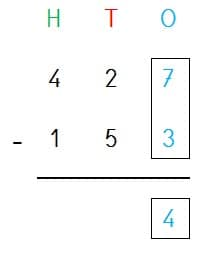1st) Can we subtract 7 - 3 in the ones column?

Yes, because 7 is greater than 3 and therefore we don't need to borrow from the tens column.

7 - 3 = 4

2nd) Can we subtract 2 - 5 in the tens column?

No, because 2 is less than 5 and, therefore, we must ask the hundreds column for help.

3rd) We reduce 1 hundred of the minuend figure (hundreds column) and we borrow it to the minuend figure of the tens column taking into account that 1 hundred equals to 10 tens:

Minuend (hundreds column):

4 hundreds - 1 hundred = 3 hundreds

Minuend (tens column):

2 tens + 10 tens (1 hundred) = 12 tens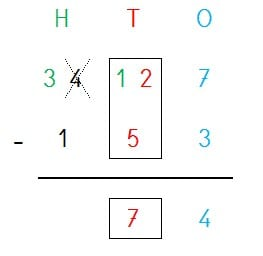4th) We subtract the tens column because 12 is greater than 5:

12 - 5 = 7

5th) Finally, we will subtract the hundreds column:

3 - 1 = 2

6th) We already have the solution!

427 - 153 = 274

Practice subtraction of numbers with eight and nine vertical digits and borrowing with whole numbers from 0 to 1,000,000,000 having fun with educational games and with exercises ready to print in PDF.#### Solve subtractions of three elements vertically without regrouping with whole numbers up to 999,999,999

How to subtract 3 or more 8 and 9-digit numbers without borrowing?

We explain step by step how to perform the calculations and how to find the result easily in the subtractions with three elements no regrouping up to 9 digits.#### Mentally solve simple subtraction facts with natural numbers up to 999,999,999

Show your logical-mathematical skills by solving our exercises with hidden or missing whole numbers and using mental calculation (also known as "mental math").#### Solve subtraction word problems without regrouping with natural numbers up to 999,999,999

We have made some exercises so that you can practice solving subtraction word problems no borrowing with the numbers from 0 to 1,000,000,000.

To make it easier for you, we provide you a step-by-step explanation through a video tutorial with 2 digit word problems without regrouping.

SPANISHENGLISH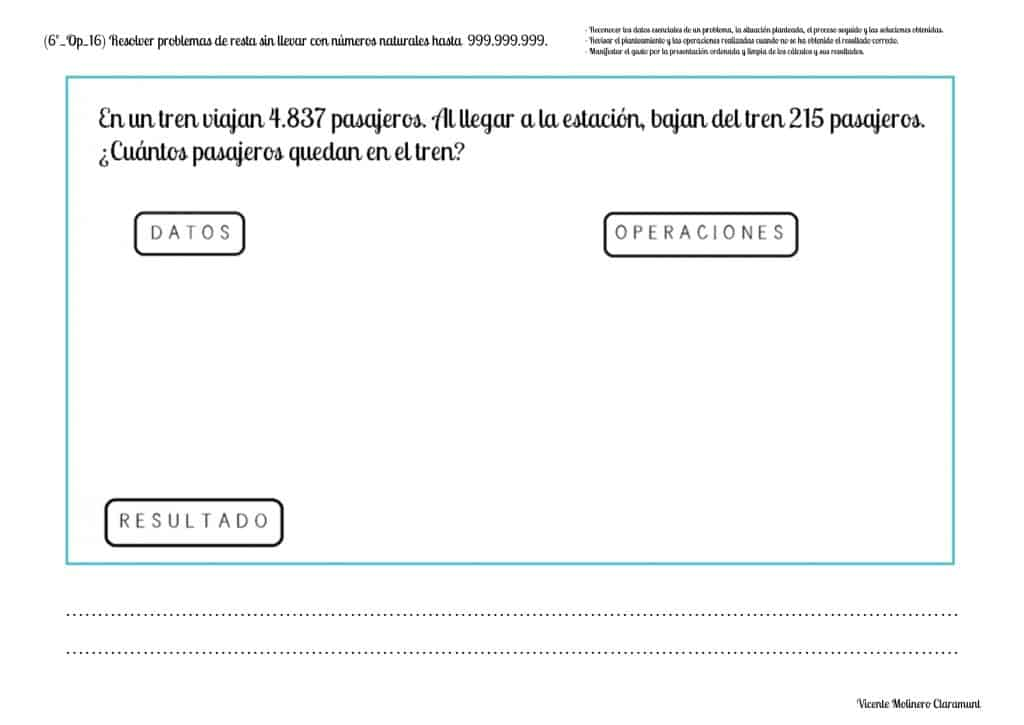#### Solve subtraction word problems with regrouping with whole numbers up to 999,999,999

Show that you are a math genius by solving each of our arithmetic problems using the basic operation of subtraction by borrowing from 0 to 1,000,000,000.

If you need a little preparation, watch our video lessons where we provide you with some solved examples using the column method.

SPANISHENGLISH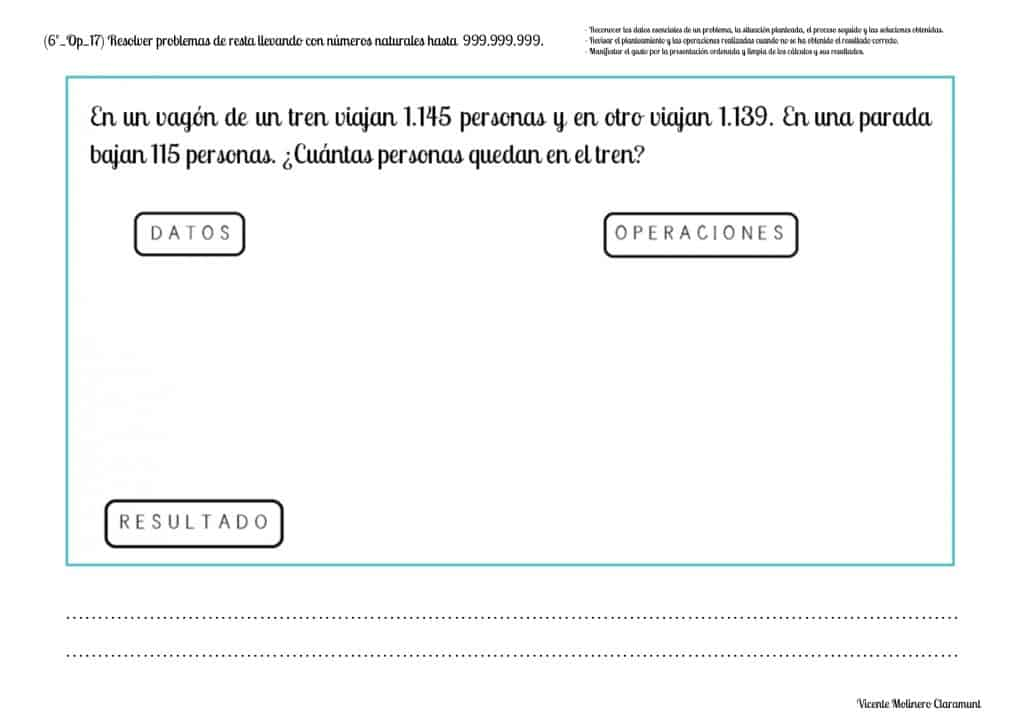#### Check the subtraction using addition to verify the accuracy of calculations

How to check if the result of a subtraction is correct?

Cheking subtraction using addition helps us recognize if we have correctly solved any subtraction.

Once we have obtained the difference (or result), we can make sure if we have subtracted correctly using the addition and applying the following mathematical formula:

Minuend = Subtrahend + Difference

SPANISHENGLISH